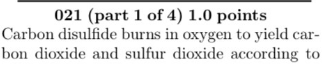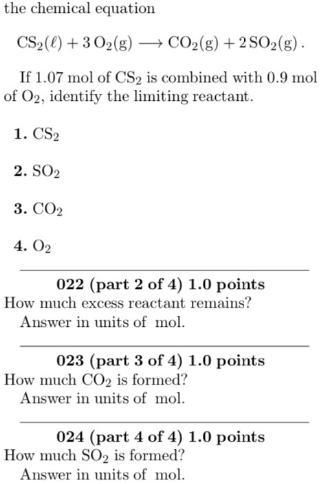# Carbon disulfide burns in oxygen to yield carbon dioxide and sulfur dioxide according to the chemical equation CS2(ℓ) + 3 O2(g) → CO2(g) + 2SO2(g) If 1.07 mol of CS2 is combined with 0.9 mol O2, identify the limiting reactant. 1. CS2 2. SO2 3. CO2 4. O2 How much excess reactant remains? Answer in units of mol. How much CO2 is formed? Answer in units of mol. How much SO2 is formed? Answer in units of mol.